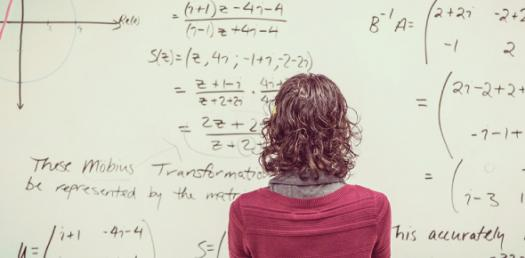# 5th Grade Basic Multiplication Quiz

10 Questions | Attempts: 4600
ShareSettingsThis is a 5th-grade basic multiplication quiz that is designed to help students that have a hard time solving such problems. Do you feel like you understand how to solve various mathematical problems involving multiplication? How about you take up this quiz and keep a look out for others like it so as to perfect your skills? All the best to you, and we hope you enjoy this quiz! Let's go now!

• 1.
If 12x12=X, then what is X?
• A.

12

• B.

143

• C.

144

• D.

179

• 2.
If 9x8=X, then what is X?
• A.

27

• B.

68

• C.

71

• D.

72

• 3.
If 3x25=X, then what is X?
• A.

75

• B.

95

• C.

99

• D.

103

• 4.
If 8x8=X, then what is X?
• A.

64

• B.

72

• C.

83

• D.

99

• 5.
If 2x12=X, then what is X?
• A.

12

• B.

24

• C.

36

• D.

48

• 6.
71x2=X, then what is X?
• A.

142

• B.

144

• C.

146

• D.

180

• 7.
If 13x9=X, then what is X?
• A.

97

• B.

101

• C.

107

• D.

117

• 8.
If 12x0=X, then what is X?
• A.

6

• B.

18

• C.

0

• D.

120

• 9.
If 7x8=X, then what is X?
• A.

12

• B.

24

• C.

54

• D.

56

• 10.
48x 16=X, then what is X?
• A.

723

• B.

768

• C.

432

• D.

728

## Related TopicsBack to top
×

Wait!
Here's an interesting quiz for you.